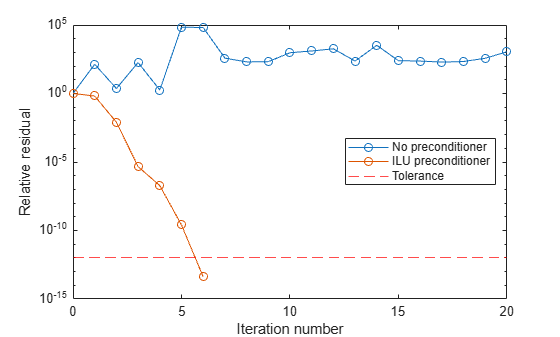Documentation

# bicg

## Syntax

```x = bicg(A,b) bicg(A,b,tol) bicg(A,b,tol,maxit) bicg(A,b,tol,maxit,M) bicg(A,b,tol,maxit,M1,M2) bicg(A,b,tol,maxit,M1,M2,x0) [x,flag] = bicg(A,b,...) [x,flag,relres] = bicg(A,b,...) [x,flag,relres,iter] = bicg(A,b,...) [x,flag,relres,iter,resvec] = bicg(A,b,...) ```

## Description

`x = bicg(A,b)` attempts to solve the system of linear equations `A*x = b` for `x`. The `n`-by-`n` coefficient matrix `A` must be square and should be large and sparse. The column vector `b` must have length `n`. `A` can be a function handle, `afun`, such that `afun(x,'notransp')` returns `A*x` and `afun(x,'transp')` returns `A'*x`.

Parameterizing Functions explains how to provide additional parameters to the function `afun`, as well as the preconditioner function `mfun` described below, if necessary.

If `bicg` converges, it displays a message to that effect. If `bicg` fails to converge after the maximum number of iterations or halts for any reason, it prints a warning message that includes the relative residual `norm(b-A*x)/norm(b)` and the iteration number at which the method stopped or failed.

`bicg(A,b,tol)` specifies the tolerance of the method. If `tol` is `[]`, then `bicg` uses the default, `1e-6`.

`bicg(A,b,tol,maxit)` specifies the maximum number of iterations. If `maxit` is `[]`, then `bicg` uses the default, `min(n,20)`.

`bicg(A,b,tol,maxit,M)` and `bicg(A,b,tol,maxit,M1,M2)` use the preconditioner `M` or `M = M1*M2` and effectively solve the system ```inv(M)*A*x = inv(M)*b``` for `x`. If `M` is `[]` then `bicg` applies no preconditioner. `M` can be a function handle `mfun`, such that `mfun(x,'notransp')` returns `M\x` and `mfun(x,'transp')` returns `M'\x`.

`bicg(A,b,tol,maxit,M1,M2,x0)` specifies the initial guess. If `x0` is `[]`, then `bicg` uses the default, an all-zero vector.

`[x,flag] = bicg(A,b,...)` also returns a convergence flag.

Flag

Convergence

`0`

`bicg` converged to the desired tolerance `tol` within `maxit` iterations.

`1`

`bicg` iterated `maxit` times but did not converge.

`2`

Preconditioner `M` was ill-conditioned.

`3`

`bicg` stagnated. (Two consecutive iterates were the same.)

`4`

One of the scalar quantities calculated during `bicg` became too small or too large to continue computing.

Whenever `flag` is not `0`, the solution `x` returned is that with minimal norm residual computed over all the iterations. No messages are displayed if the `flag` output is specified.

`[x,flag,relres] = bicg(A,b,...)` also returns the relative residual `norm(b-A*x)/norm(b)`. If `flag` is `0`, ```relres <= tol```.

`[x,flag,relres,iter] = bicg(A,b,...)` also returns the iteration number at which `x` was computed, where `0 <= iter <= maxit`.

`[x,flag,relres,iter,resvec] = bicg(A,b,...)` also returns a vector of the residual norms at each iteration including `norm(b-A*x0)`.

## Examples

### Using bicg with a Matrix Input

This example shows how to use `bicg` with a matrix input. `bicg`. The following code:

```n = 100; on = ones(n,1); A = spdiags([-2*on 4*on -on],-1:1,n,n); b = sum(A,2); tol = 1e-8; maxit = 15; M1 = spdiags([on/(-2) on],-1:0,n,n); M2 = spdiags([4*on -on],0:1,n,n); x = bicg(A,b,tol,maxit,M1,M2);```

displays this message:

```bicg converged at iteration 9 to a solution with relative residual 5.3e-009```

### Using bicg with a Function Handle

This example replaces the matrix `A` in the previous example with a handle to a matrix-vector product function `afun`. The example is contained in a file `run_bicg` that

• Calls `bicg` with the `@afun` function handle as its first argument.

• Contains `afun` as a nested function, so that all variables in `run_bicg` are available to `afun`.

Place the following into a file called `run_bicg`:

```function x1 = run_bicg n = 100; on = ones(n,1); b = afun(on,'notransp'); tol = 1e-8; maxit = 15; M1 = spdiags([on/(-2) on],-1:0,n,n); M2 = spdiags([4*on -on],0:1,n,n); x1 = bicg(@afun,b,tol,maxit,M1,M2); function y = afun(x,transp_flag) if strcmp(transp_flag,'transp') % y = A'*x y = 4 * x; y(1:n-1) = y(1:n-1) - 2 * x(2:n); y(2:n) = y(2:n) - x(1:n-1); elseif strcmp(transp_flag,'notransp') % y = A*x y = 4 * x; y(2:n) = y(2:n) - 2 * x(1:n-1); y(1:n-1) = y(1:n-1) - x(2:n); end end end```

When you enter

`x1 = run_bicg;`

MATLAB® software displays the message

```bicg converged at iteration 9 to a solution with ... relative residual 5.3e-009```

### Using bicg with a Preconditioner

This example demonstrates the use of a preconditioner.

Load `A = west0479`, a real 479-by-479 nonsymmetric sparse matrix.

```load west0479; A = west0479;```

Define `b` so that the true solution is a vector of all ones.

`b = full(sum(A,2));`

Set the tolerance and maximum number of iterations.

```tol = 1e-12; maxit = 20;```

Use `bicg` to find a solution at the requested tolerance and number of iterations.

`[x0,fl0,rr0,it0,rv0] = bicg(A,b,tol,maxit);`

`fl0` is 1 because `bicg` does not converge to the requested tolerance `1e-12` within the requested 20 iterations. In fact, the behavior of `bicg` is so poor that the initial guess (`x0 = zeros(size(A,2),1)`) is the best solution and is returned as indicated by `it0 = 0`. MATLAB® stores the residual history in `rv0`.

Plot the behavior of `bicg`.

```semilogy(0:maxit,rv0/norm(b),'-o'); xlabel('Iteration number'); ylabel('Relative residual');```The plot shows that the solution does not converge. You can use a preconditioner to improve the outcome.

Create the preconditioner with `ilu`, since the matrix `A` is nonsymmetric.

```[L,U] = ilu(A,struct('type','ilutp','droptol',1e-5)); ```
```Error using ilu There is a pivot equal to zero. Consider decreasing the drop tolerance or consider using the 'udiag' option. ```

MATLAB cannot construct the incomplete LU as it would result in a singular factor, which is useless as a preconditioner.

You can try again with a reduced drop tolerance, as indicated by the error message.

```[L,U] = ilu(A,struct('type','ilutp','droptol',1e-6)); [x1,fl1,rr1,it1,rv1] = bicg(A,b,tol,maxit,L,U);```

`fl1` is 0 because `bicg` drives the relative residual to `4.1410e-014` (the value of `rr1`). The relative residual is less than the prescribed tolerance of `1e-12` at the sixth iteration (the value of `it1`) when preconditioned by the incomplete LU factorization with a drop tolerance of `1e-6`. The output `rv1(1)` is `norm(b)`, and the output `rv1(7)` is `norm(b-A*x2)`.

You can follow the progress of `bicg` by plotting the relative residuals at each iteration starting from the initial estimate (iterate number 0).

```semilogy(0:it1,rv1/norm(b),'-o'); xlabel('Iteration number'); ylabel('Relative residual');```## References

 Barrett, R., M. Berry, T.F. Chan, et al., Templates for the Solution of Linear Systems: Building Blocks for Iterative Methods, SIAM, Philadelphia, 1994.